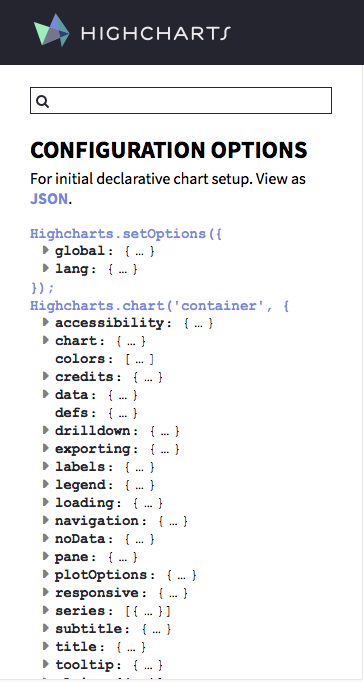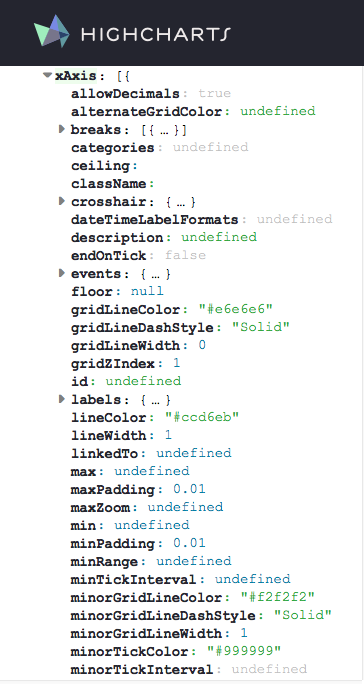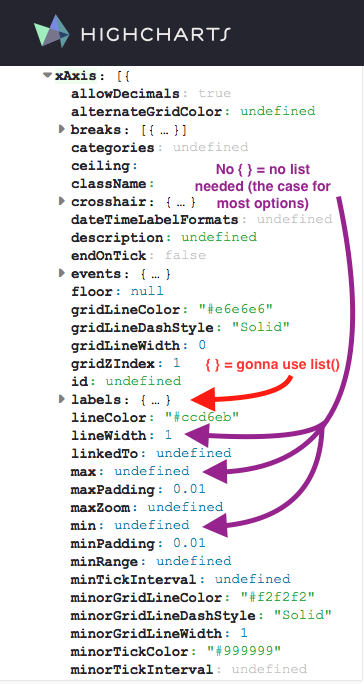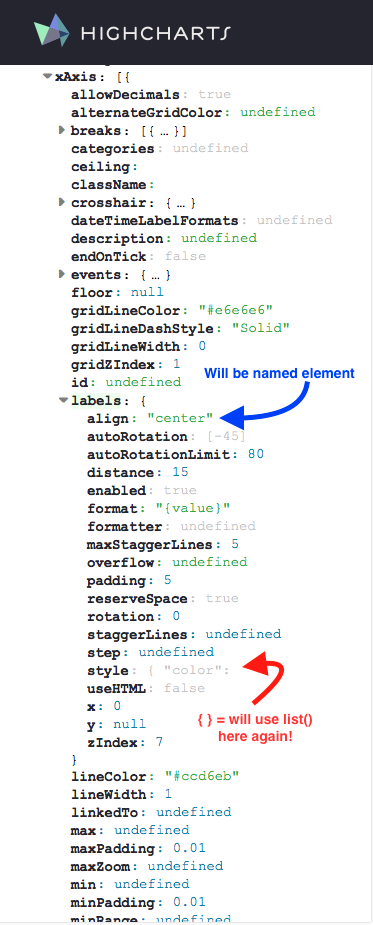Rstudio’s Mine Cetinkaya-Rundel had a post about the `highcharter` package, a wrapper for the Highcharts javascripts library that lets you create super sweet interactive charts in R.

Joshua Kunst’s `highcharter` package has become my go-to plotting package once I reach the production phase and know I will be using HTML. This is mainly for 3 reasons:

1. Beautiful interactive charts
2. Extremely customizable
3. Great documentation (via Highcharts API). Requires understanding how a `highcharter` object is built and translating between the Highcharts the API.

`ggplot2` is wonderfully customizable and the `plotly` wrapper can make interactive charts. I’m sure that `plotly` objects are customizable, as are other `htmlwidget` graphing packages, but I think Highcharts graphs are the most impressive. Hence, `highcharter` is where I have decided to dig deep.

As Mine noted, all products in this library are free for non-commercial use. If you plan to use `highcharter` in `R` for commericial use, please purchase Highcharts: https://shop.highsoft.com/

Also, a special thanks to Joshua Kunst for developing this package. I’ve learned a lot about `R` and `javascript` thanks to you. Y, gracias a ti mi amigo, siempre estaré empleado.

## Goal of this Post

My goal is to show you how I think about, learn about, and then build more complicated `highcharter` objects in `R`. I want you to be able to see a graph on the Highcharts demo page and think to yourself, “yeah, I can build that”. This will means some bouncing between the Highcharts demo and API websites. I do this a lot myself and I hope, by the end of this document, you’ll find it a useful habit.

But I also want to say that my approach to building plots in `highcharter` can feel a bit complicated. Generally, I like to build plots from the ground up. However, if I have tidy data and I know the structure is well suited for plotting in `highcharter`, I will opt to use the `hchart()`. Otherwise, for almost anything more complicated, I will build the data structure (known as a “series”) from scratch and use the `highchart()` and `hc_add_series_list()` functions. This process is the result after having built many, many plots, hacking away at the great many functions in `highcharter`. While it may seem complicated, I assure you that it is the easiest and cleanest way to make complicated plots.

## What I will not cover

• Straightforward, simple plotting using `highcharter`. Mine’s post and Joshua Kunst’s online documentation are better resources for that. I will use simple examples, but as a stepping stone to more complicated plots and to learn how to “translate” Highcharts to `highcharter`.
• How to make Highstocks or Highmaps using `highcharter`. That said, the way I go about building Highcharts plot will likely translate over to Highstocks and Highmaps, so this post may prove useful if you’re interested in making timeseries or map plots.

## Prerequisites

This post assumes that you have a good handle of the `tidyverse` as well as basic object and list construction. In short, this is not a beginner’s tutorial but also not an advanced `R` tutorial.

# Series: `highcharter` and Highcharts building blocks

Series are the building blocks of a Highcharts plot. Series contain the sets of data you want to plot. As a data scientist who wants to use `highcharter` to make spiffy plots, the key to building any plot in `highcharter` is understanding how to build a series in `R` and how it relates to the structure of a series in Highcharts.

I learn best by example. I will do the same in this document.

## Series in `highcharter` are a list of lists with a specific structure

Think of any series you would like to plot in `highcharter` as a list of lists. The Highcharts equivalent is, at the very least, an array of with a single data object or, a its most complicated, an array of many objects and arrays.

Here is a simple Highcharts plot:

``````\$(function () {
Highcharts.chart('container', {
series: [{
data: [29.9, 71.5, 106.4, 129.2, 144.0, 176.0, 135.6, 148.5, 216.4, 194.1, 95.6, 54.4]
}]
});
});``````

Ignore everything but `series`. A series array (`series: [ ]`) with a single series object (`{data: [ ] }`) is the simplest Highcharts plot possible. Translated to `R`, a series would be a list with a single sublist with named elements. Note that “named elements” means I explicitly assign the elements of a list to a value i.e. unnamed elements `list(c(1,2,3))` vs named `list(x = c(1,2,3))`. The named element in this case is `data` resulting in list structure `list(data = c( ))`.

``````series = list(
list(
data = c(29.9, 71.5, 106.4, 129.2, 144.0, 176.0, 135.6, 148.5, 216.4, 194.1, 95.6, 54.4)
)
)``````

This series can be plotted using `highchart()` and `hc_add_series_list()`

``````library(highcharter)
library(tidyverse)

highchart() %>%
hc_chart(backgroundColor = "white") %>%

By default, `highchart()` assumes you are construction a Highcharts line chart. It also provides default series names (i.e. `Series 1`, `Series 2` etc) and colors if these values are left unspecified.

Let’s change the series name and color to `'Hola linea'` and `'red'`. In Highcharts, the series array object would look like this:

``````\$(function () {
Highcharts.chart('container', {
series: [{
name: 'Hola linea',
color: 'red',
data: [29.9, 71.5, 106.4, 129.2, 144.0, 176.0, 135.6, 148.5, 216.4, 194.1, 95.6, 54.4]
}]
});
});``````

Translated to `R`, the series would be

``````series = list(
list(
name = 'Hola linea',
color = 'red',
data = c(29.9, 71.5, 106.4, 129.2, 144.0, 176.0, 135.6, 148.5, 216.4, 194.1, 95.6, 54.4)
)
)
highchart() %>%
hc_chart(backgroundColor = "white") %>%

Let’s add a second series. The series array object now contains two series. Each series is an object (contain in `{ }`, separated by `,`) with named elements (aka “members”).

``````\$(function () {
Highcharts.chart('container', {
series: [{
name: 'Hola linea',
color: 'red',
data: [29.9, 71.5, 106.4, 129.2, 144.0, 176.0, 135.6, 148.5, 216.4, 194.1, 95.6, 54.4]
},
{ // there's a comma between objects in { }
name: 'Reverse!',
color: 'green',
data: [54.4, 95.6, 194.1, 216.4, 148.5, 135.6, 176, 144, 129.2, 106.4, 71.5, 29.9]
}]
});
});``````

And in R

``````series = list(
list(
name = 'Hola linea',
color = 'red',
data = c(29.9, 71.5, 106.4, 129.2, 144.0, 176.0, 135.6, 148.5, 216.4, 194.1, 95.6, 54.4)
),
list(
name = 'Reverse!',
color = 'green',
data = c(54.4, 95.6, 194.1, 216.4, 148.5, 135.6, 176, 144, 129.2, 106.4, 71.5, 29.9)
)
)

highchart() %>%
hc_chart(backgroundColor = "white") %>%

Important: Naming matters. The Highcharts API for line series explicitly looks for object elements like `name`, `color`, `data` etc. Likewise, this means that the element names when building lists in `R` also matter. Try changing `data` to `datas` (or anything else) and see that nothing will be plotted. Likewise, try changing `name` to something else like `nombres`, and the series will fall back to the default `Series #`.

### `hc_add_series_list()` vs `hc_add_series()`

I prefer to always use `hc_add_series_list()`, even when only add a single series. Adding a single series can be done using `hc_add_series()`. For example, I could replicated the last plot by layering one series at a time.

``````highchart() %>%
hc_chart(backgroundColor = "white") %>%
name = 'Hola linea',
color = 'red',
data = c(29.9, 71.5, 106.4, 129.2, 144.0, 176.0, 135.6, 148.5, 216.4, 194.1, 95.6, 54.4)
) %>%
name = 'Reverse!',
color = 'green',
data = c(54.4, 95.6, 194.1, 216.4, 148.5, 135.6, 176, 144, 129.2, 106.4, 71.5, 29.9)
)``````

Notice that the construction of `hc_add_series()` is basically equivalent to how I built each list object in `series` that was then passed to `hc_add_series_list()`. I prefer, however, building list objects and saving them to a value (like `series`). This makes it easier to reuse the object and also makes for much shorter pipe chains when plotting.

## Highcharts API and `highcharter` functions

### Just throw an `hc_` infront of it

Now that we’ve build a basic plot and have some understanding of what a series is, let’s play with some plot options!

The beauty of the `highcharter` package is that pratically every Highcharts API call can be quickly translated to `highcharter` without needing to look at `highcharter` documentation. Specifically, any Highcharts API options can be access by add `hc_` infront of the function e.g. `hc_xAxis()` calls the `xAxis` API option, `hc_tooltip()` calls the `tooltip` API option, etc.

Here is what I mean. When you go to the Highcharts API Options Reference, there is a menu of list of “main” option calls to the left. It looks like this:The `highcharter` equivalent to each “main” option can be accessed as a function by throwing an `hc_` infront (`chart` becomes `hc_chart()`, `plotOptions` becomes `hc_plotOptions()` etc).

From there, accessing any “main” option value means using the exact same name as listed in the API. Any level deeper just means contructing a `list()` but the API reference names will always be the same.

Let’s work through an example by editing the x-axis looking only at the Highcharts API x-axis options.If I want to change the min, max, the line width, and some labeling quirks of the x-axis, then I just look at the API options for `xAxis` and locate the corresponding values.

In this case, three of these suboptions (`min`, `max`, `lineWidth`) are “unnested” level options, one is a “nested” level option (`labels`). What I call an “unnested” level suboption make up the majority of suboptions—any without `{ ... }`, easily found by the little gray expansion triangle. “Unnested” suboptions can be accessed by using the unnested level API names asis plus a proper value. “Nested” level suboptions—those that are followed by `{...}` or just any `{`—require `list(...)` calls.In the image above, examples of “unnested” level suboptions (i.e. suboptions with no `{...}`; access without a list) are in purple. One need only use the API name and provide a proper value. Examples of “nested” level suboptions (i.e. suboptions with `{ ... }`; require biulding a `list(...)` object) are in red.

Knowing the “main” API option I want to use is `xAxis`, I can build the `highcharter` equivalent by throwing an `hc_` infront. I can then directly call any of the “unnested” level suboptions `min`, `max`, and `lineWidth` since they are not nested `{ ... }` objects. Using only the “unnested” level calls, the result would be `hc_xAxis(min = 1, max = 7, lineWith = 5)`.

Note: As I said before, elements names must exactly match the API names, meaning suboptions are case-sensitive (i.e. `linewidth` \(\ne\) `lineWidth`).

But what about nested level calls which require lists? The nested level call I cared about was `labels`. Expanding the API main option, the `labels` suboptions nests numerous more suboptions. One of them, `style`, is another nested suboption—the value starts with `{ ...`. Again—and hopefully you’re starting to see the pattern!—`style` suboption values can be accessed by building a named `list()`.I choose two of the `labels` suboptions to change: `align` and `style`. `align` isn’t nested so I can just assign the proper value. The default is `"center"`. I change it to `"left"`: `align = "left"`.

But `style` is another nested suboption (valuestarts with `{`). But again, not to worry, this just means another `list()`. I’ll change the font size, weight, and color style values: `style = list(fontSize = "16px", fontWeight = "bold", color = "blue")`. The nested fully constructed `labels` suboption is:

``````labels = list(align = "left",
style = list(
fontSize = "16px",
fontWeight = "bold",
color = "blue"
))``````

I can then add this `labels` as aother `hc_xAxis()` suboption:

``````hc_xAxis(min = 1,
max = 7,
lineWith = 5,
labels = list(align = "left",
style = list(
fontSize = "16px",
fontWeight = "bold",
color = "blue"
)))``````

Throwing this all together, I can adjust the x-axis of the plot above by adding my fully constructed `hc_xAxis` function to the pipe chain.

``````highchart() %>%
hc_chart(backgroundColor = "white") %>%
hc_xAxis(min = 1,
max = 7,
lineWith = 5,
labels = list(align = "left",
style = list(
fontSize = "16px",
fontWeight = "bold",
color = "blue"
)))``````

The corresponding code in `Highcharts` can be see here.

## Use `hchart()` with Tidy Data

One of the most convinient function for plotting is the `hchart()` function. But I would only recommend the use of this function if one has a Tidy Dataframe structured in a “long” format with a time-key-value or key-value structure, similar to a dataframe that would be used in `ggplot`. I’ll show you what I mean.

Here is some code from an example in `highcharter` that builds a graph by extracting variables from `citytemp` and adding them as a series.

``````data(citytemp)
hc1 <- highchart() %>%
hc_chart(backgroundColor = "white") %>%
hc_xAxis(categories = citytemp\$month) %>%
hc_add_series(name = "Tokyo", data = citytemp\$tokyo) %>%
hc_add_series(name = "London", data = citytemp\$london)
hc1``````

`citytemp` is in a “wide” format. But this data isn’t tidy. In these data, there are three variables: `month`, `city`, `temperature`. In this case, `month` is time, `city` is a key, and `temperature` is a value. I want to reshape the data such that each row of data is a single observation for the temprature of one city at one point in time. Reshaping the data to a “long” format with a tidy time-key-value structure will allow us to plot virtually the same plot but in one line using `hchart()`.

``````citytemp2 <- citytemp %>%
tidyr::gather(key = city, value = temperature, tokyo, london)``````

I can now use the `hchart()` function to plot these data. How the data splits into separate series is via the `group` variable in the `hchart()` function. Notice that the mapping of variables uses the function `highcharter::hcaes()`, which was inspired by the `ggplot2` function `ggplot2::aes()` and has the same syntax.

``````hchart(citytemp2, type = 'line', hcaes(y = temperature, group = city, x = month)) %>%
hc_chart(backgroundColor = "white")``````

If I just wanted to print data for tokyo and london, I can just filter the data prior to using `hchart()`.

``````citytemp2 <- citytemp2 %>%
dplyr::filter(city %in% c('tokyo', 'london')) # filter to just tokyo and london

hc2 <- hchart(citytemp2, type = 'line', hcaes(y = temperature, group = city, x = month))
hc2 %>%
hc_chart(backgroundColor = "white")``````

NOTE: whatever is passed as the `x` variable must generally be of class `Date`, `character` or `numeric`. Other types aren’t handled nicely, like class `yearmon` from the `zoo` package. Often, the best strategy is to order the data, NOT pass a `x` variable, then label the x-axis later using the `hc_xAxis(categories = some_vector_of_strings)` option.

# Your Best Friend, the `hc\$x\$hc_opts\$series` List

Pretend you assigned your chart to the variable `hc`. You can extract the underlying series data used in the chart by digging into the lists `hc\$x\$hc_opts\$series`. Referencing these series list is actually how I learned to finally start connecting the Highcharts API with tooltip options and series construction.

But this is where things also start to get tricky. How you pass data to `highcharter` or how you build a series affects the underlying structure of the data used for plotting. I will again show this by example.

Below, I build two charts with essentially the same output, `hc1` and `hc2`. `hc1` is built series by series, explicitly defining the series `name` and `data` (remember, the list names `name` and `data` are explicit, matching the API calls). `hc2` is built series by passing a tidy dataframe to `hchart`, defining the `x` and `y` values but letting the series names be defined by the `group` variable.

``````# build series by series
hc1 <- highchart() %>%
hc_chart(backgroundColor = "white") %>%
hc_xAxis(categories = citytemp\$month) %>%
hc_add_series(name = "tokyo", data = citytemp\$tokyo) %>%
hc_add_series(name = "london", data = citytemp\$london)
hc1``````
``````# build using hchart
citytemp2 <- citytemp %>%
tidyr::gather(key = city, value = temperature, tokyo, london)

hc2 <- hchart(citytemp2, type = 'line', hcaes(y = temperature, group = city, x = month))
hc2 %>%
hc_chart(backgroundColor = "white")``````

So what? If the output is the same, what is there to fret about? Enter `.\$x\$hc_opts\$series`.

Here is the underlying structure of the data used to make the `hc1` plot.

``length(hc1\$x\$hc_opts\$series)``
``#>  2``
``hc1\$x\$hc_opts\$series # here is the series data and metadata``
``````#> []
#> []\$data
#>    7.0  6.9  9.5 14.5 18.2 21.5 25.2 26.5 23.3 18.3 13.9  9.6
#>
#> []\$name
#>  "tokyo"
#>
#>
#> []
#> []\$data
#>    3.9  4.2  5.7  8.5 11.9 15.2 17.0 16.6 14.2 10.3  6.6  4.8
#>
#> []\$name
#>  "london"``````
``hc1\$x\$hc_opts\$series[] # i can extract a specific series``
``````#> \$data
#>    7.0  6.9  9.5 14.5 18.2 21.5 25.2 26.5 23.3 18.3 13.9  9.6
#>
#> \$name
#>  "tokyo"``````
``hc1\$x\$hc_opts\$series[]\$data # also just the plotting data, which is a vector in this case``
``#>    7.0  6.9  9.5 14.5 18.2 21.5 25.2 26.5 23.3 18.3 13.9  9.6``

`hc1\$x\$hc_opts\$series` is therefore a list of lists, where each element of the list `.\$series` contains the plotting data. The structure of the data in this case is pretty simple and clean.

But now let’s look at `hc2`.

There series count is the same (`2`). But I’m not going to display the output of `hc2\$x\$hc_opts\$series` because it is crazy long. However, I recommend running the code `hc2\$x\$hc_opts\$series` yourself in the console and taking a look.

``length(hc2\$x\$hc_opts\$series)``
``#>  2``
``````# hc2\$x\$hc_opts\$series # commented out because its so long
hc2\$x\$hc_opts\$series[][["name"]] # notice how we are extracting from the second series, "tokyo", not the first! Why is that? See the "Important Note" below.``````
``#>  "tokyo"``
``hc2\$x\$hc_opts\$series[]\$data %>% head(2) # extract the first 2 elements of the data for the tokyo series``
``````#> []
#> []\$month
#>  "Jan"
#>
#> []\$new_york
#>  -0.2
#>
#> []\$berlin
#>  -0.9
#>
#> []\$city
#>  "tokyo"
#>
#> []\$temperature
#>  7
#>
#> []\$y
#>  7
#>
#> []\$name
#>  "Jan"
#>
#>
#> []
#> []\$month
#>  "Feb"
#>
#> []\$new_york
#>  0.8
#>
#> []\$berlin
#>  0.6
#>
#> []\$city
#>  "tokyo"
#>
#> []\$temperature
#>  6.9
#>
#> []\$y
#>  6.9
#>
#> []\$name
#>  "Feb"``````

IMPORTANT NOTE: Why is the `tokyo` data the second series in `hc2`, which used `hchart`, but the first series in `hc1`? This is because, when you build the chart series by series (using `hc_add_series`), the index follows the order of inclusion. The `tokyo` data is added to `hc1` chart first, so it becomes the first series (`.\$series[]`). On the other hand, if you use `hchart`, then highcharter orders series alphabetically. `london` comes before `tokyo`, so `tokyo` is the second series (`.\$series[]`). Given that the order of how a series is plotted can matter, this is a very important caveat to remember when using `hchart`!

The fact that `hc2\$x\$hc_opts\$series` is so much longer than the very concise `hc1\$x\$hc_opts\$series` is telling you that `hchart` is a very different beast than building using `hc_add_series` or `hc_add_series_list`. Yet, despite having very different underlying series structures, each produces the same visual output.

What’s going on? Why is the structure so different?

Let’s dive deeper by looking at the first element of the `data` list for the `tokyo` series in `hc1` and `hc2`. Remember, the `tokyo` data is the first series in `hc1` and the second in `hc2`.

``````# first data element of tokyo series
hc1\$x\$hc_opts\$series[]\$data[] # first series here (ordered by how series was added)``````
``#>  7``
``hc2\$x\$hc_opts\$series[]\$data[] # but second series here (ordered alphabetically by series name)``
``````#> \$month
#>  "Jan"
#>
#> \$new_york
#>  -0.2
#>
#> \$berlin
#>  -0.9
#>
#> \$city
#>  "tokyo"
#>
#> \$temperature
#>  7
#>
#> \$y
#>  7
#>
#> \$name
#>  "Jan"``````

For reference, here, again, is that code that generated `hc1` and `hc2`. I also recommend looking at the structure of the `citytemp` and `citytemp2` data objects to refresh yourself.

``````hc1 <- highchart() %>%
hc_chart(backgroundColor = "white") %>%
hc_xAxis(categories = citytemp\$month) %>%
hc_add_series(name = "tokyo", data = citytemp\$tokyo) %>%
hc_add_series(name = "london", data = citytemp\$london)

hc2 <- hchart(citytemp2, type = 'line', hcaes(y = temperature, group = city, x = month))``````

The value of `hc1\$x\$hc_opts\$series[]\$data[]` is `7`. This maps directly with `citytemp\$tokyo`. This is because the data for each series in `hc1` is a single vector (or array) of data (`..., data = citytemp\$tokyo)`). A nice chart is still generated because the API handles the translation of the 1d `data` vector to `y` values and creates the corresponding `x` index values.

But the value of `hc2\$x\$hc_opts\$series[]\$data[]` is a list of 5 named elements.

``hc2\$x\$hc_opts\$series[]\$data[] %>% str()``
``````#> List of 7
#>  \$ month      : chr "Jan"
#>  \$ new_york   : num -0.2
#>  \$ berlin     : num -0.9
#>  \$ city       : chr "tokyo"
#>  \$ temperature: num 7
#>  \$ y          : num 7
#>  \$ name       : chr "Jan"``````

The best way to think of element `hc2\$x\$hc_opts\$series[]\$data[]` is as a point with, in this case, 5 bits of data. While each bit of data exists as part of the point, not all of the data is used when creating the chart. The only bits of data used are those that have names used by the Highcharts API, like `y` and `name`.

There are a few things to notice, each rooted in the code `hchart(., type = 'line', hcaes(y = temperature, group = city, x = month))`:

1. The dataframe `citytemp2` had only 3 variables (`month`, `city`, `temperature`). `hchart` not only passes these variables as data, it then passes the variables assigned to the `y` and `x` arguments (`y = temperature`, `x = month`).
2. But wait, there is no `x` named element? The variable assigned to `x` was renamed to `name`, *which is not to be confused with the series name `hc2\$x\$hc_opts\$series[]\$name`. This is because the variable assigned to `x` was of class `character`. Highcharts cannot chart a non-numeric value to the x-axis. Instead, `highcharter` automatically maps string values assigned to `x` to the list element `name`. Index values are then generated for `x` by the API and each is labeled by the `name` value.

### One-dimensional array? Easy! Multi-dimensional arrays? Damn.

Turns out that Highcharts, by default, parses the contents of the `data` array object element by element, looking for sub-arrays with specific names (`y`, `x` etc) or a specific order (`[2, 5]` order implies `x = 2`, `y = 5`).

If Highcharts finds a single unlabeled `data` array instead of an array with subarray elements (essentially a one-dimensional vector vs a list of lists), it assumes that the data maps to `y` and creates an index for each value to act as the x-axis. That is, in the background, the API takes the single 1-dim array, assumes its the data for `y`, and then creates `(x, y)` array pairs where `x` is just an index `1:length(y)`.

Things start getting cumbersome and confusing the moment you want to chart anything more complicated than a one-dimensional vector/array—like specific `(x,y)` data pairs or perhaps extra data to label specific points or to make fancy tooltips. In `R` terms, this means that `data` goes from being a simple vector to a sprawling list of sublists where each element of `data` (e.g. `data[]`) is actually another named list.

The pros of this is you have total control over what you want to plot! The downside is that a simple change to a chart can sometimes be incredibly tedious.

If you just want a plot with no special customizations, no problem! Easy, clean, straightforward. But the moment you want to customize even one point in a series, the amount and complexity of code you have to write just ballooned 2 or 3 fold.

My next post will cover how to deal with these more complex plots. It can be tedious but the results are beautiful. As I told my old boss, “It is amazing how much you have to code to make there be less in a plot.”

Danton Noriega

4256 Words

2017-04-04 17:00 -0700